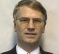## Matlab Basic Functions Reference (pdf)Started by 3 years ago6 replieslatest reply 3 years ago212 views

Matlab has posted a pdf document that lists basic functions.  Here is a link to the pdf.

-- Neil

[ - ][ - ]Hi Neil.

Thanks for providing the link to the useful PDF file.

[ - ]After 30 years Mathworks gets it tidy. Better than never. I think they have copied it from a third party user as I have seen similar tables in a 2008 book.

[ - ]It would be nice if they also included a note/clarification about the row/column, X-Y, angle conventions for 'row vectors' etc.

The conventions can confuse many, especially for graphs when 2d image processing!

[ - ]True row/column could be confusing. I am not sure what you mean by X-Y, angle...etc

Here are notes from an old post of mine:

The terminology of rows/columns of a matrix can be confusing.

Matlab(and hence Octave) views x(r,c) as r = number of rows, c =  number of columns.

however,visually r then indicates number of samples per column and c number of samples per row.

Matlab(and Octave) functions work columnwise i.e. all samples in one column are viewed as one vector.

[ - ]